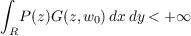#### Vol. 92, No. 1, 1981

 Recent Issues Vol. 307: 1  2 Vol. 306: 1  2 Vol. 305: 1  2 Vol. 304: 1  2 Vol. 303: 1  2 Vol. 302: 1  2 Vol. 301: 1  2 Vol. 300: 1  2Online Archive Volume: Issue:The Journal Editorial Board Subscriptions Officers Special Issues Submission Guidelines Submission Form Contacts ISSN: 1945-5844 (e-only) ISSN: 0030-8730 (print) Author Index To Appear Other MSP Journals
The equations Δu = Pu (P 0) on Riemann surfaces and isomorphisms between relative Hardy spaces

### Takeyoshi Satō

Vol. 92 (1981), No. 1, 173–194
##### Abstract

It has been demonstrated by M. Nakai that the Banach spaces PB (the space of bounded solutions on R of the equation Δu = Pu, P 0) and HB (the space of bounded harmonic functions on R) are isometrically isomorphic whenever the conditionis valid for some point w0 in R (z = x + iy). Here, G(z,w) is the harmonic Green’s function on R. In this paper we shall show, under the preceding condition that the Hardy space Hp, 1 < p +, of harmonic functions on a hyperbolic Riemann surface R is isometrically isomorphic to the relative Hardy space PHwp of quotients of solutions of Δu = Pu by the P-elliptic measure w of R.

##### Mathematical Subject Classification 2000
Primary: 30F15
Secondary: 30F20, 31A35
##### Milestones